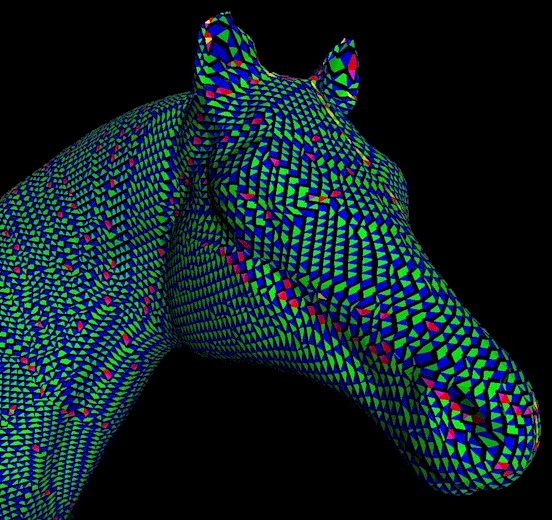Having my way with Ulysses

# Hush, I hear feetstoops12:16 am

Ok, stoop down here.  Don’t mind me, just picking up these racing forms. Figure this out on my own.  La la la. So what do we have.  Sceptre 5 to 4, Zinfandel 7 to 4, Maximum II 10 to 1.  Those are the most likely.  Will include least likely, Throwaway 20 to 1 for a control.  A throwaway.  So.  Ok.  Draw a circle, homogeneous non-differentiated space.  Divide into duality in order to create.  Division within unity.  Now.  Center is O and radius OA=1.  Diameters AA’ and BB’ at right angles and with centers on diameter BB’ draw two circles.  Ok,  each with a radius half that of original circle.  Yup. From point A swing an arc NM tangent to circumferences of the two inner circles.  Repeat from point A’.  Construct square ACB’O from the radius of the original circle.  That’s the racetrack.  Good.  Ok.  The arc of the semi-diagonal of the square and the radius AE of the arc NEM is Φ  and the arcs NEM and NDM divide the radii AO and A’O into the golden division of 1/Φ and 1/Φ2.  Hum. Paradox now. Divide a circle into a yin yang like that and the circumferences of the inner circles are equal to that of the larger circle but the area within them is only half that of the original circle. One has become two. So Zinfandel?  Not Maximum II.  That seems out.   A unity becomes a duality.   Homogeneity becomes polarized.  Separated.  Jockey falls off?  Which one?  Mutually repellent forms arise from a common source.  Well that’s life.  And that sounds like Zinfandel to me.  The pentahedron to the cube, the heptahedron to the cube, the decahedron to the unity.  The icosahedron to the unity.  Hm.  So now, construct a square equal in area to the original circle.  If only pi were 3.17, then it would be Maximum II and what a payoff!  But have to base this on reality.  So.  So.  Φ2= 1+r2 and r = √Φ-1 and r = √Φ and the circumference equals 2∏√Φ with √Φ=1.272. . . and ∏=three point one four one five nine do da do da, so, hm.  If the perimeter of the square is approximately 8.  But I don’t want approximates.  Then, well.  This isn’t very mathematically exact.  A bit like throwing money into a hurricane.  But it looks like Sceptre.  That’s just where it’s shaking out.  I can feel it in the numbers.  My money, all of it, on Scepter.  Respect.  A dead cert.